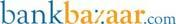Rediff.com  » Business » How daily interest on savings bank a/cs is calculated

How daily interest on savings bank a/cs is calculated

April 12, 2010 17:27 ISTFrom April 1, 2010, interest on all savings bank account deposits is being calculated on a daily basis, thereby earning account holdres higher interest income. This is due to the fact that the Reserve Bank of India has instructed banks to change the mechanism of interest income calculation. The calculation is done on the 'daily balance method', with the rate of interest remaining the same at 3.5% p.a.

Earlier method of interest rate calculation

Earlier, banks would pay interest at the rate of 3.5% p.a. on the lowest available balance in the account between the tenth and the last day of the month.

Any deposit in the account between the tenth and the end of the month, would not earn the account holder any interest as it is not part of the interest rate calculation.

Any withdrawal between the same period would result in lower interest income as the lowest balance would be taken into account for the calculation.

Example: Ashwin had an account balance of Rs 85,000 on April 10. He received a payment of Rs 300,000 on April 15 from the sale of some mutual fund units.

On April 29, he made a down payment of Rs 320,000 to a builder for a property. This resulted in his account balance reducing to Rs 65,000. For the interest income calculation for the month of April, the bank would take Rs 65,000 as the base and pay him interest on that amount. So interest due to Ashwin would be on Rs 65,000 for 30 days @ 3.5% p.a. which would be Rs 187.

In spite of having a high account balance for most period of the month, Ashwin lost interest income for the month.

Under this method of interest rate calculation, the best thing Ashwin could do is ensure that all transactions are done between the first and ninth of any month so that he would get benefit of interest. This required proper planning.

New method of interest rate calculation

Interest will be paid @3.5% p.a. on the daily balance in the account at the end of the day. Here, the account holder will get interest on the actual day end balance.

Under this method, Ashwin's interest income calculation would be:

• For the first 14 days of April,  interest to be paid would be calculated on Rs 85,000;
• For the next 14 days of April, interest to be paid would be calculated on Rs 385,000 and;
• For the balance 2 days, interest to be paid would be calculated on Rs 65,000.

So the total interest due to Ashwin would be Rs 643.

Under this method, Ashwin's interest income is higher by Rs 456!

Besides, he did not have to plan his withdrawals and deposits as he would receive interest on the actual account balance.

As a savings bank account holder, you should be pleased with the latest change. Who would not like to see higher balance on account of higher interest income?

PS: Interest rate calculation Formula

Daily interest = Amount (Daily balance) * Interest (3.5/100) / days in the year.

BankBazaar.com

Powered byBankBazaar.com is an online marketplace where you can instantly get loan rate quotes, compare and apply online for your personal loan, home loan and credit card needs from India's leading banks and NBFCs.